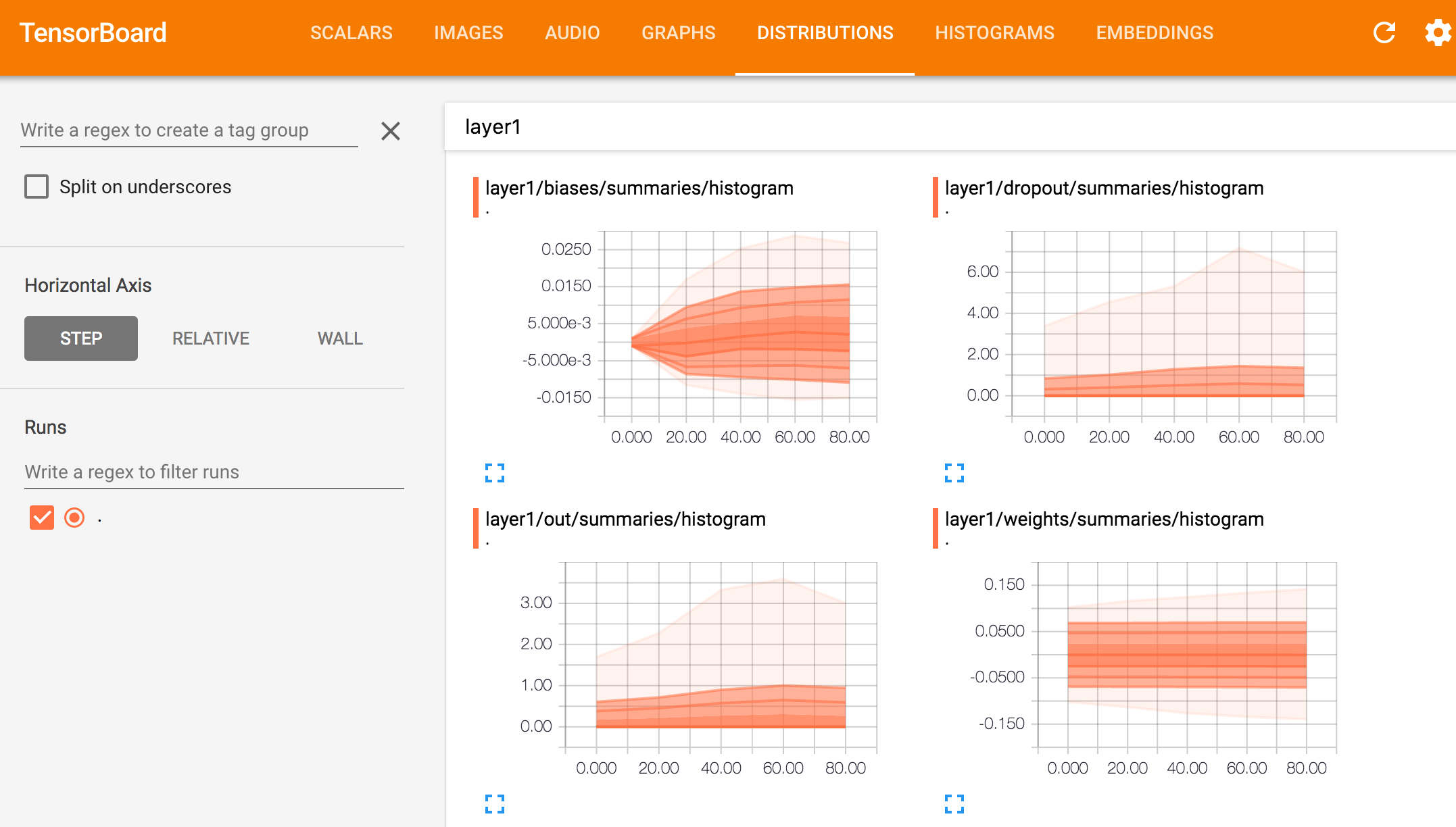### TensorBoard

TensorBoard is a browser based application that helps you to visualize your training parameters (like weights & biases), metrics (like loss), hyper parameters or any statistics. For example, we plot the histogram distribution of the weight for the first fully connected layer every 20 iterations.#### Namespace

To create some data hierarchy structure when we view the data like: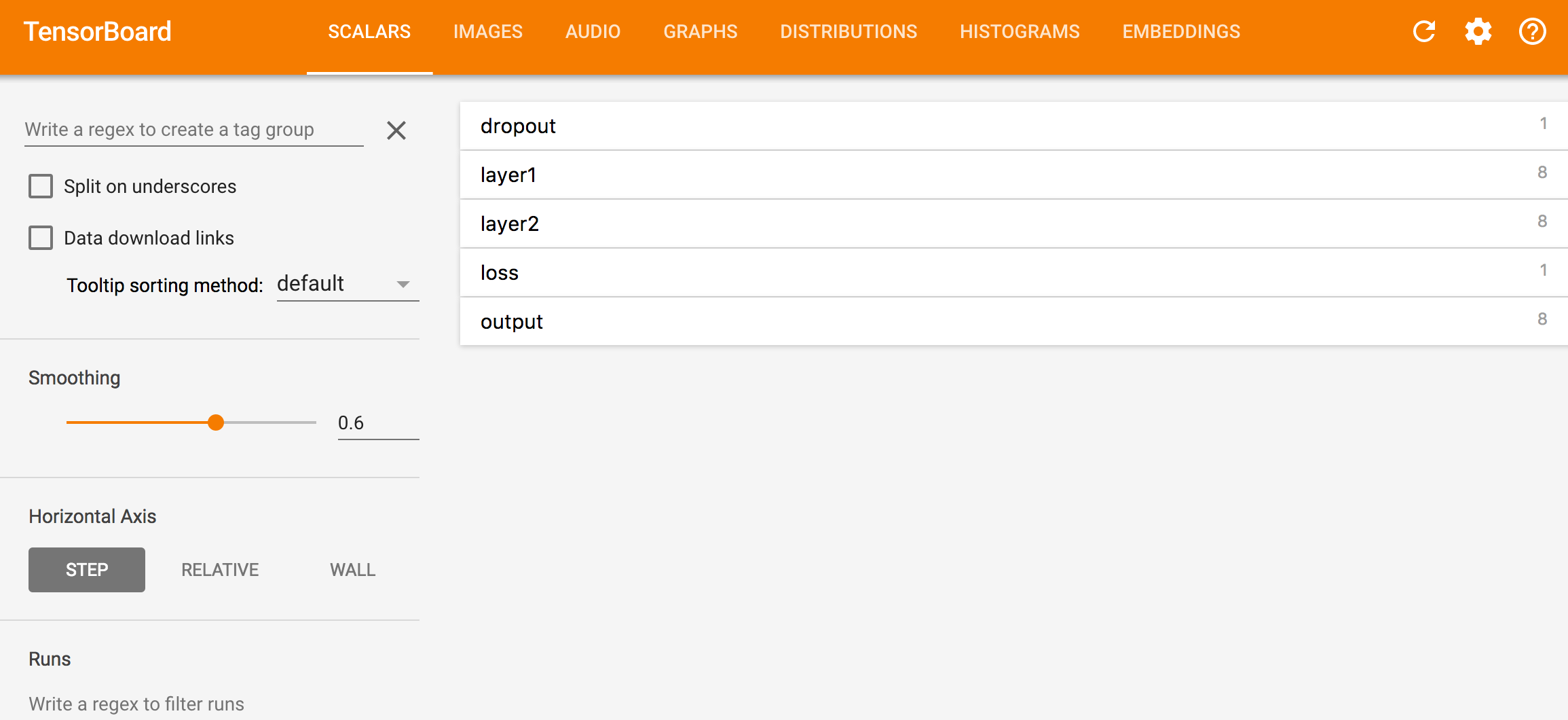We use namespace

with tf.name_scope('CNN1'):
with tf.name_scope('W'):
mean = tf.reduce_mean(W)
tf.summary.scalar('mean', mean)
stddev = tf.sqrt(tf.reduce_mean(tf.square(W - mean)))
tf.summary.scalar('stddev', stddev)
tf.summary.histogram('histogram', var)


Which create the following data hierarchy and can be browsed with the TensorBoard later: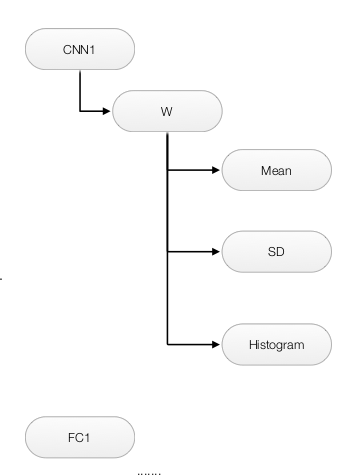#### Implement TensorBoard

To add & view data summaries to the TensorBoard. We need to:

1. Define all the summary information to be logged.
2. Add summary information to the writer to flush it out to a log file.
3. View the data in the TensorBoard.

### Define summary information

Number can be added to the TensorBoard with tf_summary.scalar and array with tf.summary.histogram

with tf.name_scope('CNN1'):
with tf.name_scope('W'):
mean = tf.reduce_mean(W)
tf.summary.scalar('mean', mean)
tf.summary.histogram('histogram', var)


For data logged with tf_summary.scalar. The accuracy increases while cost drops.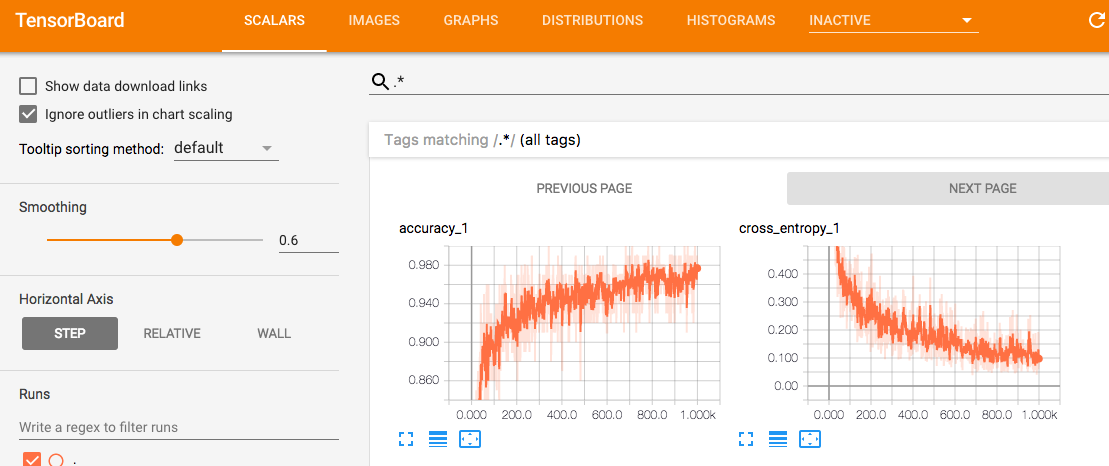More statistics on the weights for layer 1.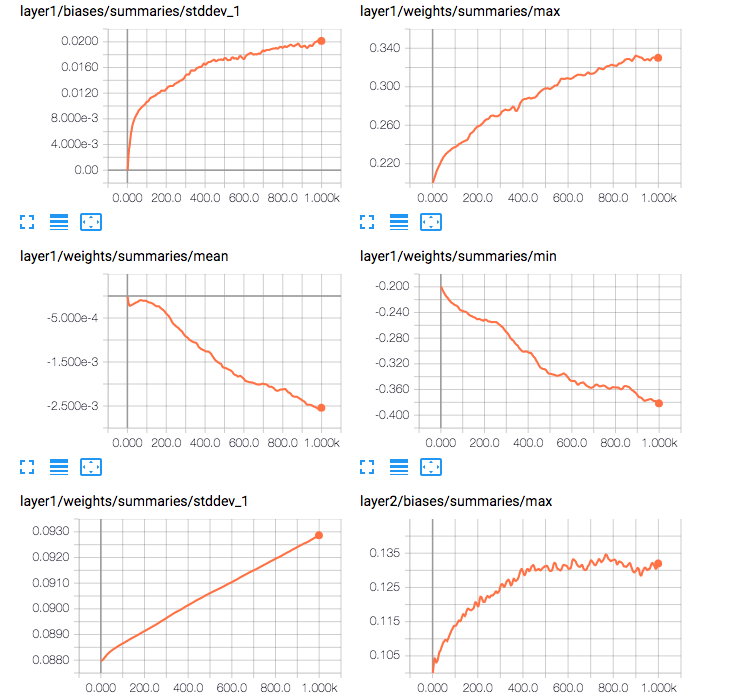For summary logged with tf.summary.histogram#### Example

Here is a more complicated example in which we try to summarize the information of the weight in the first fully connected layer.

h1, _ = affine_layer(x, 'layer1', [784, 256], keep_prob)

def affine_layer(x, name, shape, keep_prob, act_fn=tf.nn.relu):
with tf.name_scope(name):
with tf.name_scope('weights'):
W = tf.Variable(tf.truncated_normal(shape, stddev=np.sqrt(2.0 / shape)))
variable_summaries(W)

def variable_summaries(var):
with tf.name_scope('summaries'):
mean = tf.reduce_mean(var)
tf.summary.scalar('mean', mean)
with tf.name_scope('stddev'):
stddev = tf.sqrt(tf.reduce_mean(tf.square(var - mean)))
tf.summary.scalar('stddev', stddev)
tf.summary.scalar('max', tf.reduce_max(var))
tf.summary.scalar('min', tf.reduce_min(var))
tf.summary.histogram('histogram', var)


### Add summary information to a writer

After we define what summary information to be logged, we merge all the summary data into one single operation node with tf.summary.merge_all(). We create a summary writer with tf.summary.FileWriter, and then write and flush out the information to the log file every 20 iterations:

def main(_):
...

# Merge all summary inforation.
summary = tf.summary.merge_all()

init = tf.global_variables_initializer()
with tf.Session() as sess:

# Create a writer for the summary data.
summary_writer = tf.summary.FileWriter(FLAGS.log_dir, sess.graph)

sess.run(init)
for step in range(100):
...
sess.run(train_step, feed_dict={x: batch_xs, labels: batch_ys, lmbda:5e-5, keep_prob:0.5})
if step % 20 == 0:
# Write summary
summary_str = sess.run(summary, feed_dict={x: batch_xs, labels: batch_ys, lmbda:5e-5, keep_prob:0.5})
summary_writer.flush()
...


### Multimodal distributions

We can concatenate 2 Tensors to be plotted together in the same graph:

normal_combined = tf.concat([mean_moving_normal, variance_shrinking_normal], 0)
tf.summary.histogram("normal/bimodal", normal_combined)


### Plot training and validation loss/accuracy

We use the same tensor to compute the loss for the training samples and validation samples. To plot the same tensor with different datasets together, Barzin provides a solution using 2 file writers:

import tensorflow as tf
from numpy import random

writer_1 = tf.summary.FileWriter("./logs/plot_1")
writer_2 = tf.summary.FileWriter("./logs/plot_2")

log_var = tf.Variable(0.0)
tf.summary.scalar("loss", log_var)

write_op = tf.summary.merge_all()

session = tf.InteractiveSession()
session.run(tf.global_variables_initializer())

for i in range(100):
# for writer 1
summary = session.run(write_op, {log_var: random.rand()})
writer_1.flush()

# for writer 2
summary = session.run(write_op, {log_var: random.rand()})
writer_2.flush()


### View the TensorBoard

Open the terminal and run the Tensorboard command with you log file location.

\$ tensorboard --logdir=/tmp/tensorflow/mnist/log
Starting TensorBoard b'41' on port 6006
(You can navigate to http://192.134.44.11:6006)


We can add runtime performance information into the TensorBoard

      merged = tf.summary.merge_all()
...

run_options = tf.RunOptions(trace_level=tf.RunOptions.FULL_TRACE)
summary, _ = sess.run([merged, train_step],
feed_dict=feed_dict(True),
options=run_options,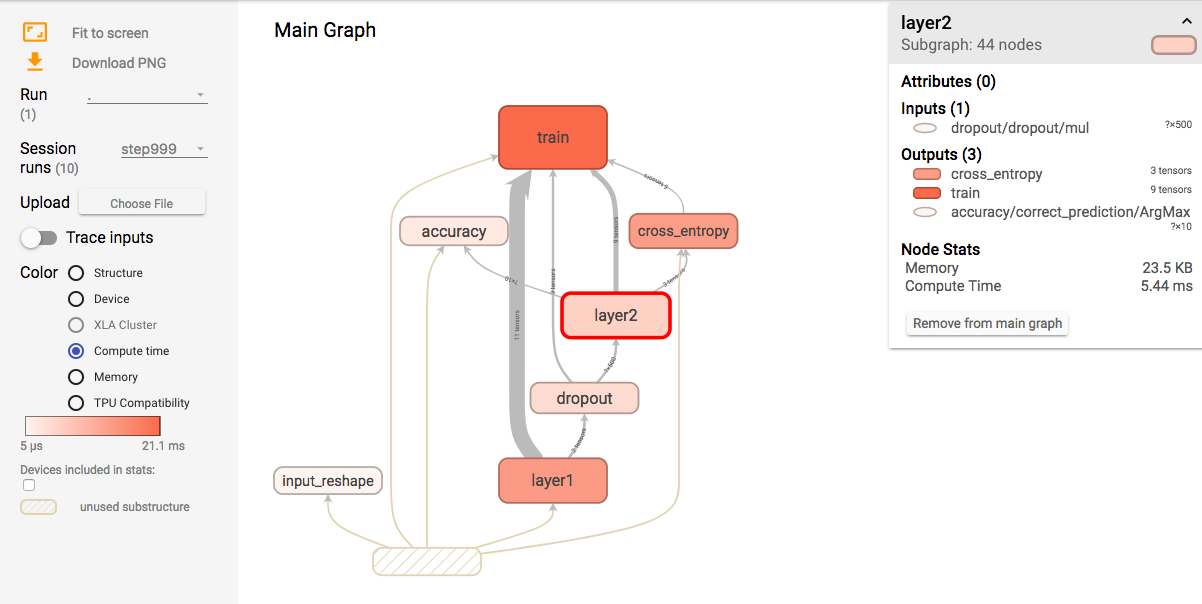### Full program listing

# Copyright 2015 The TensorFlow Authors. All Rights Reserved.
#
# you may not use this file except in compliance with the License.
# You may obtain a copy of the License at
#
#
# Unless required by applicable law or agreed to in writing, software
# WITHOUT WARRANTIES OR CONDITIONS OF ANY KIND, either express or implied.
# See the License for the specific language governing permissions and
# ==============================================================================
"""A simple MNIST classifier which displays summaries in TensorBoard.
This is an unimpressive MNIST model, but it is a good example of using
tf.name_scope to make a graph legible in the TensorBoard graph explorer, and of
naming summary tags so that they are grouped meaningfully in TensorBoard.
It demonstrates the functionality of every TensorBoard dashboard.
"""
from __future__ import absolute_import
from __future__ import division
from __future__ import print_function

import argparse
import os
import sys
z
import tensorflow as tf

from tensorflow.examples.tutorials.mnist import input_data

FLAGS = None

def train():
# Import data
one_hot=True,
fake_data=FLAGS.fake_data)

sess = tf.InteractiveSession()
# Create a multilayer model.

# Input placeholders
with tf.name_scope('input'):
x = tf.placeholder(tf.float32, [None, 784], name='x-input')
y_ = tf.placeholder(tf.float32, [None, 10], name='y-input')

with tf.name_scope('input_reshape'):
image_shaped_input = tf.reshape(x, [-1, 28, 28, 1])
tf.summary.image('input', image_shaped_input, 10)

# We can't initialize these variables to 0 - the network will get stuck.
def weight_variable(shape):
"""Create a weight variable with appropriate initialization."""
initial = tf.truncated_normal(shape, stddev=0.1)
return tf.Variable(initial)

def bias_variable(shape):
"""Create a bias variable with appropriate initialization."""
initial = tf.constant(0.1, shape=shape)
return tf.Variable(initial)

def variable_summaries(var):
"""Attach a lot of summaries to a Tensor (for TensorBoard visualization)."""
with tf.name_scope('summaries'):
mean = tf.reduce_mean(var)
tf.summary.scalar('mean', mean)
with tf.name_scope('stddev'):
stddev = tf.sqrt(tf.reduce_mean(tf.square(var - mean)))
tf.summary.scalar('stddev', stddev)
tf.summary.scalar('max', tf.reduce_max(var))
tf.summary.scalar('min', tf.reduce_min(var))
tf.summary.histogram('histogram', var)

def nn_layer(input_tensor, input_dim, output_dim, layer_name, act=tf.nn.relu):
"""Reusable code for making a simple neural net layer.
It does a matrix multiply, bias add, and then uses ReLU to nonlinearize.
It also sets up name scoping so that the resultant graph is easy to read,
and adds a number of summary ops.
"""
# Adding a name scope ensures logical grouping of the layers in the graph.
with tf.name_scope(layer_name):
# This Variable will hold the state of the weights for the layer
with tf.name_scope('weights'):
weights = weight_variable([input_dim, output_dim])
variable_summaries(weights)
with tf.name_scope('biases'):
biases = bias_variable([output_dim])
variable_summaries(biases)
with tf.name_scope('Wx_plus_b'):
preactivate = tf.matmul(input_tensor, weights) + biases
tf.summary.histogram('pre_activations', preactivate)
activations = act(preactivate, name='activation')
tf.summary.histogram('activations', activations)
return activations

hidden1 = nn_layer(x, 784, 500, 'layer1')

with tf.name_scope('dropout'):
keep_prob = tf.placeholder(tf.float32)
tf.summary.scalar('dropout_keep_probability', keep_prob)
dropped = tf.nn.dropout(hidden1, keep_prob)

# Do not apply softmax activation yet, see below.
y = nn_layer(dropped, 500, 10, 'layer2', act=tf.identity)

with tf.name_scope('cross_entropy'):
# The raw formulation of cross-entropy,
#
# tf.reduce_mean(-tf.reduce_sum(y_ * tf.log(tf.softmax(y)),
#                               reduction_indices=))
#
# can be numerically unstable.
#
# So here we use tf.nn.softmax_cross_entropy_with_logits on the
# raw outputs of the nn_layer above, and then average across
# the batch.
diff = tf.nn.softmax_cross_entropy_with_logits(labels=y_, logits=y)
with tf.name_scope('total'):
cross_entropy = tf.reduce_mean(diff)
tf.summary.scalar('cross_entropy', cross_entropy)

with tf.name_scope('train'):
cross_entropy)

with tf.name_scope('accuracy'):
with tf.name_scope('correct_prediction'):
correct_prediction = tf.equal(tf.argmax(y, 1), tf.argmax(y_, 1))
with tf.name_scope('accuracy'):
accuracy = tf.reduce_mean(tf.cast(correct_prediction, tf.float32))
tf.summary.scalar('accuracy', accuracy)

# Merge all the summaries and write them out to
# /tmp/tensorflow/mnist/logs/mnist_with_summaries (by default)
merged = tf.summary.merge_all()
train_writer = tf.summary.FileWriter(FLAGS.log_dir + '/train', sess.graph)
test_writer = tf.summary.FileWriter(FLAGS.log_dir + '/test')
tf.global_variables_initializer().run()

# Train the model, and also write summaries.
# Every 10th step, measure test-set accuracy, and write test summaries
# All other steps, run train_step on training data, & add training summaries

def feed_dict(train):
"""Make a TensorFlow feed_dict: maps data onto Tensor placeholders."""
if train or FLAGS.fake_data:
xs, ys = mnist.train.next_batch(100, fake_data=FLAGS.fake_data)
k = FLAGS.dropout
else:
xs, ys = mnist.test.images, mnist.test.labels
k = 1.0
return {x: xs, y_: ys, keep_prob: k}

for i in range(FLAGS.max_steps):
if i % 10 == 0:  # Record summaries and test-set accuracy
summary, acc = sess.run([merged, accuracy], feed_dict=feed_dict(False))
print('Accuracy at step %s: %s' % (i, acc))
else:  # Record train set summaries, and train
if i % 100 == 99:  # Record execution stats
run_options = tf.RunOptions(trace_level=tf.RunOptions.FULL_TRACE)
summary, _ = sess.run([merged, train_step],
feed_dict=feed_dict(True),
options=run_options,
else:  # Record a summary
summary, _ = sess.run([merged, train_step], feed_dict=feed_dict(True))
train_writer.close()
test_writer.close()

def main(_):
if tf.gfile.Exists(FLAGS.log_dir):
tf.gfile.DeleteRecursively(FLAGS.log_dir)
tf.gfile.MakeDirs(FLAGS.log_dir)
train()

if __name__ == '__main__':
parser = argparse.ArgumentParser()
default=False,
help='If true, uses fake data for unit testing.')
help='Number of steps to run trainer.')
help='Initial learning rate')
help='Keep probability for training dropout.')
'--data_dir',
type=str,
default=os.path.join(os.getenv('TEST_TMPDIR', '/tmp'),
'tensorflow/mnist/input_data'),
help='Directory for storing input data')
'--log_dir',
type=str,
default=os.path.join(os.getenv('TEST_TMPDIR', '/tmp'),
'tensorflow/mnist/logs/mnist_with_summaries'),
help='Summaries log directory')
FLAGS, unparsed = parser.parse_known_args()
tf.app.run(main=main, argv=[sys.argv] + unparsed)

# 0.9816


### TensorBoard images & embedding

TensorFlow can also plot many different kinds of information including images and word embedding. To add image summary:

image = tf.reshape(x[:1], [-1, 28, 28, 1])
tf.summary.image("image", image)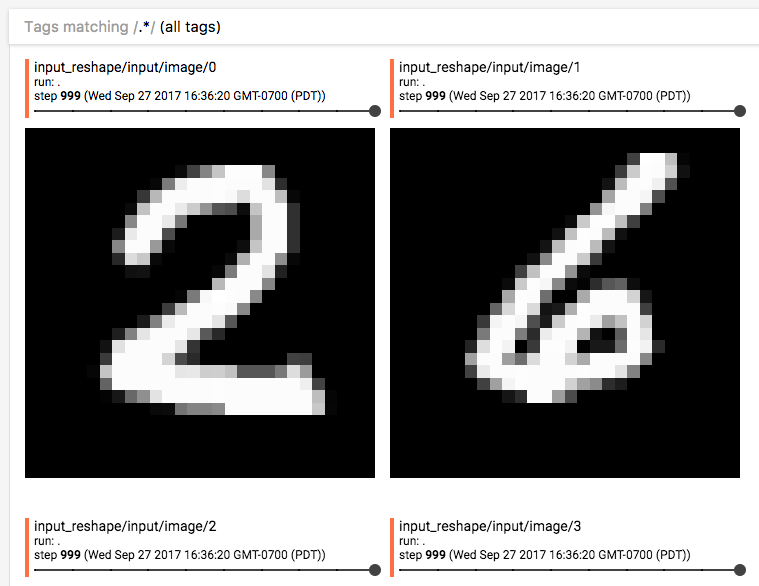Image source from TensorFlow: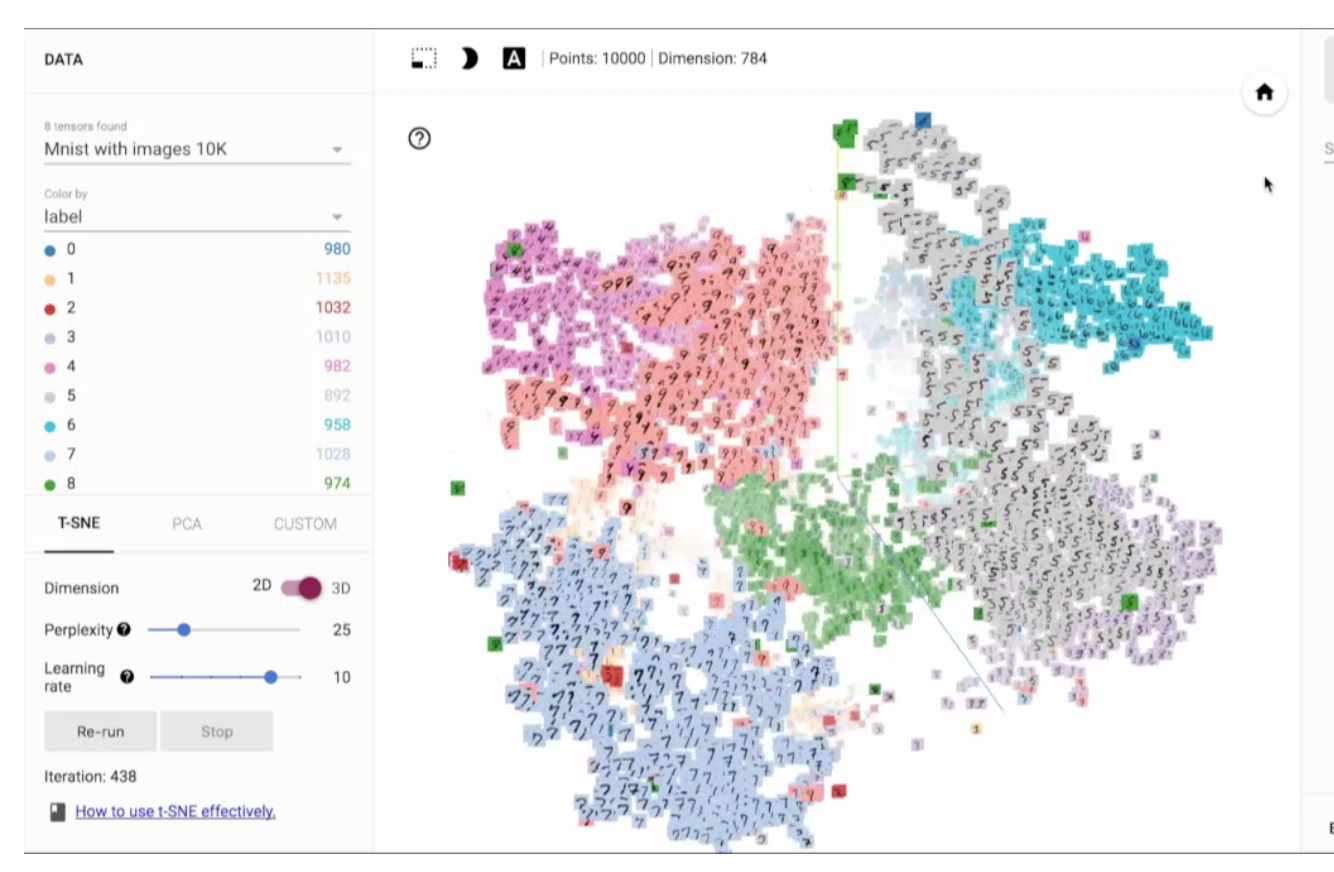### TensorBoard graph & distribution

TensorFlow automatically plots the computational graph and can be viewed under the graph tab.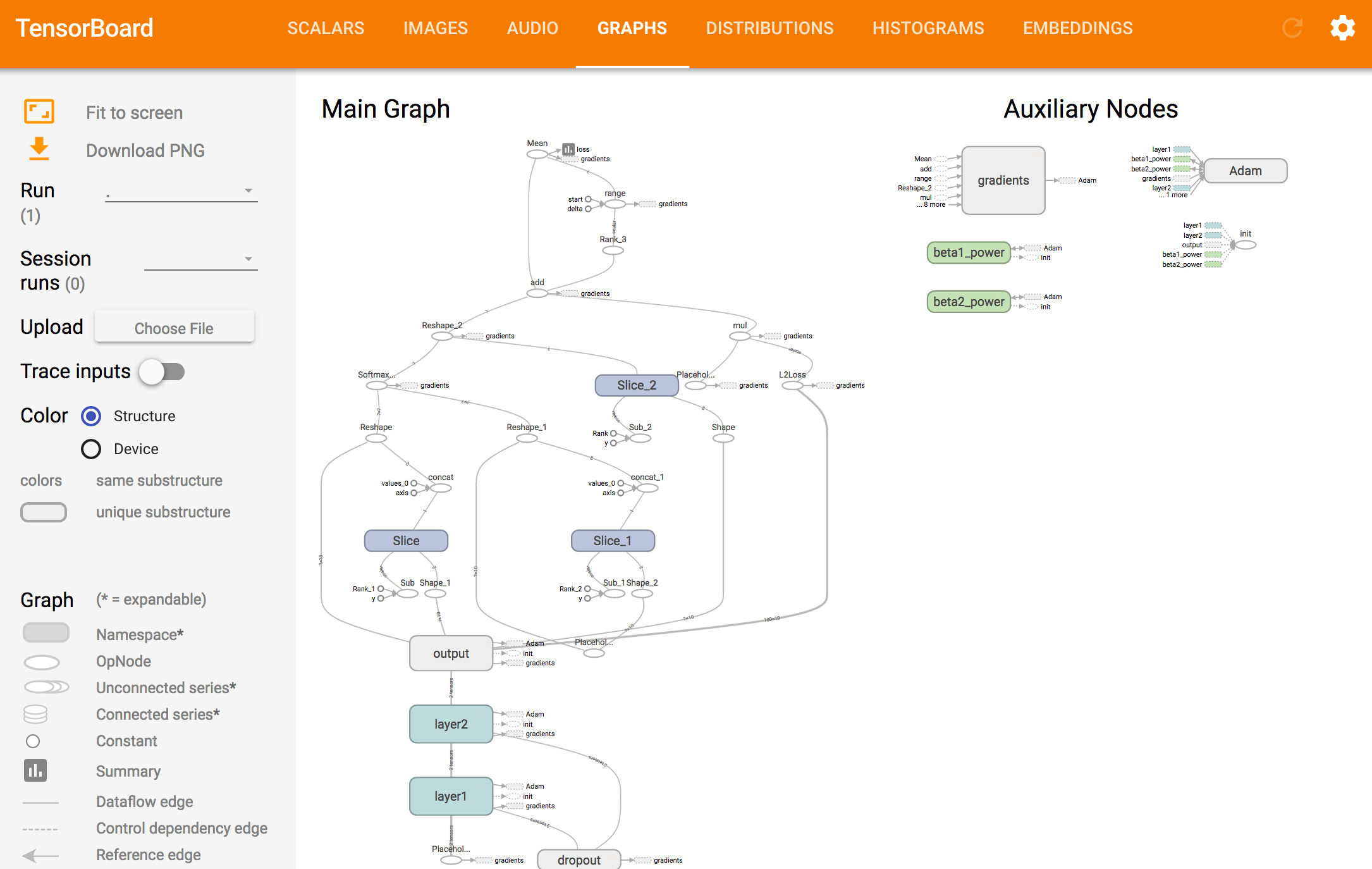All histogram can also view as distribution.